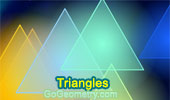# Triangles: Theorems and Problems 13Geometry Problem 624
Triangle, Incenter, Excenter, Incircle, Excircle, Similarity.

Geometry Problem 623
Triangle, Incenter, Excenter, Incircle, Excircle, Concyclic Points.

Geometry Problem 621
Three Rectangles, Diagonals, Centers, Angles.

Geometry Problem 620
Three Rectangles, Diagonals, Centers, Angles.

Geometry Problem 619
Triangle Area, 30 Degrees, Incircle, Incenter, Tangent.

Geometry Problem 618
Triangle Area, 60 Degrees, Incircle, Incenter, Tangent.

Geometry Problem 617
Right Triangle Area, Incircle, Incenter, Tangent, 90 Degrees.

Geometry Problem 616
Parallelogram, Diagonals, Intersecting Lines, Parallel Lines.

Geometry Problem 615
Right Triangle with a Square, Diagonals, Center, Area of Quadrilateral.

Geometry Problem 614
Parallelogram, Diagonals, Intersecting Lines, Parallel Lines.

Geometry Problem 613
Altitude of a Triangle, Perpendicular, Collinear Points.

Geometry Problem 612
Altitude of a Triangle, Perpendicular, Congruence.

Geometry Problem 611
Altitude of a Triangle, Perpendicular, Collinear Points, Parallel.

Geometry Problem 609
Triangle, Perpendiculars, 90 Degrees, Collinearity.

Geometry Problem 608
Square with Right Triangles, Metric Relations, Congruence, Measurement.

Geometry Problem 607
Intersecting Circles, Triangles, Metric Relations, Measurement.

Geometry Problem 606
Isosceles Triangles, Perpendicular and Parallel Lines.

Geometry Problem 604
Parallelograms, Triangle, Trapezoid, Area.

Geometry Problem 602
Triangle, Angles, 30 and 45 Degrees, Cevian, Congruence.

Geometry Problem 597
Quadrilateral, Right Triangle, Isosceles, Angle, Congruence.

Go to Page: Previous | 1 | 2 | 3 | 4 | 5 | 6 | 7 | 8 | 9 | 10 | 11 | 12 | 13 | 14 | 15 | 16 | 17 | 18 | 19 | 20 | 21 | 22 | 23 | 24 | 25 | 26 | 27 | 28 | 29 | 30 | 40 | Next

 Home | Search | Geometry | Polygon | Email | By Antonio Gutierrez